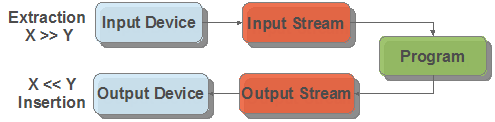# C++ Stream Operator Support

In standard C, basic I/O are handled by functions like getc(), putc() and printf() and the formatting of data is handled by functions like atoi(), atof(), strotul() and sprintf(). For example, reading a floating point number from the user over RS232 would require a combination of gets() followed by atof(). While CCS includes an input.c library that accomplishes many of these tasks, the input.c library uses a fixed RS232 stream and does not work with Keypad/LCD or USB without modification. Starting with V5 of the CCS C Compiler, CCS has added support for the C++ stream operator. C++ streams provided a unified interface for I/O and data formatting.

The two new operators added are the extraction operator and the insertion operator:

Operator Symbol Operator Name
>> Extraction
<< Insertion

When used, these operators show the direction of data. For example:The beauty of these operators is that the x and y in the above examples can be any combination of function, RS232 serial stream, variable, string and more.

One of the key features of this new feature in the CCS C Compiler is the way it automatically handles the conversion based upon the data types of the variables passed. These conversions are done automatically, no other helper functions like atof() need to be called. For example, if a variable is of float type the compiler will properly convert it from string to float on an input or convert float to string on an output.

Using C++ stream operator for output

stream << identifier

stream in the above example can be one of the following:

• cout - maps to the default #use rs232() stream. This provides compatibility for using existing C++ code that explicitly uses the cout stream class.
• RS232 stream name - A stream identified with the stream=x option of #use rs232().
• char array (string) - Data is parsed from identifier and saved to the char array with a null terminator.
• function - A function that takes a char for it's input variable. For example, lcd_putc() in CCS's lcd.c driver or usb_cdc_putc() in CCS's usb_cdc.h driver.

identifier can be can be an int, float, fixed point decimal, int1 (boolean) or char array.

identifier can be any of the following manipulators:

• hex - When converting variable to string, convert it to hex format characters (similar to %x in printf()).
• dec (default) - When converting variable to string, convert it to decimal format characters (similar to %d in printf())
• setprecision(x) - set number of places after the decimal point.
• setw(x) - set number of characters output for numbers
• boolalpha - output int1 as "true" or "false"
• noboolalpha (default) - output int1 as "1" or "0"
• fixed (default) - floating point numbers displayed in decimal format (similar to %f in printf())
• scientific - floating point numbers displayed in E notation (similar to %e in printf())
• iosdefault - all modifiers back to default settings
• endl - output CR/LF

Here is an example usage of this operator:

cout << "Price is \$" << setw(4) << setprecision(2) << cost*num << endl;

This example transmits "Price is \$" followed by the result of cost*num with two decimal places follwed by the CR/LF. This is transmitted using cout, which is the default RS232 stream.

Here is a change to the above example to display on the LCD using the lcd_putc() function provided in CCS's lcd.c driver:

lcd_putc << "Price is \$" << setw(4) << setprecision(2) << cost*num << endl;

Here is a change to the above example to save the formatting to a string variable called result_string:

result_string << "Price is \$" << setw(4) << setprecision(2) << cost*num << endl;

Using C++ stream operator for input

stream >> identifier

stream in the above example can be one of the following:

• cin - maps to the default #use rs232() stream. This provides compatibility for using existing C++ code that explicitly uses the cout stream class.
• RS232 stream name - A stream identified with the stream=x option of #use rs232().
• function - A function that returns a char. For example usb_cdc_getc() in CCS's usb_cdc.h driver. This function is called for each character, until a r is received.

identifier can be a variable that is integer, char, char array, float or fixed point integer type. Float type formats can use the E format.

identifier can be any of the following manipulators:

• hex - hex format numbers
• dec (default) - decimal format numbers
• strspace - allow spaces to be input into strings
• nostrspace (default) - spaces terminate string entry
• iosdefault - all manipulators to default settings.

Here is an example of reading a number from the user and saving it to the variable value:

cout << "Enter Number";

cin >> value;

The above example can be quickly modified to read from the USB virtual COM port using the routines in CCS's usb_cdc.h driver:

usb_cdc_putc << "Enter Number";

usb_cdc_getc >> value;

Several values can be read at a time:

cin << variable1 << variable2 << variable3;

In the above example, the input operator would stop reading into variable1 and start reading into variable2 once a character is received that is not valid for that data type. For instance, if variable1 and variable2 are both int, it would stop reading into variable1 and start reading into variable2 upon the reception of any character that isn't "0" to "9", like a space or new-line.

Data conversion from a string to a variable can also be achieved. This example converts the str string variable to the val variable. The type of conversion is determined by the data type of val:

str >> val;